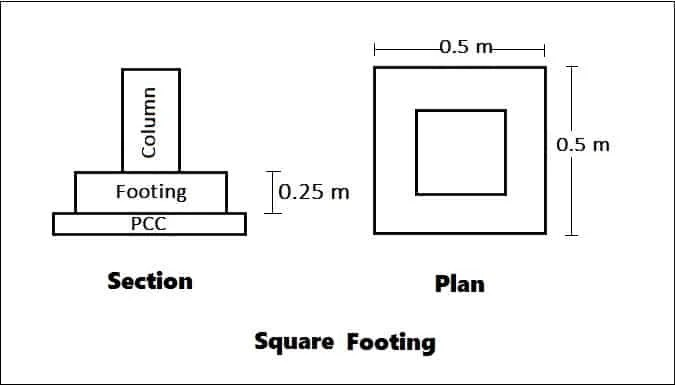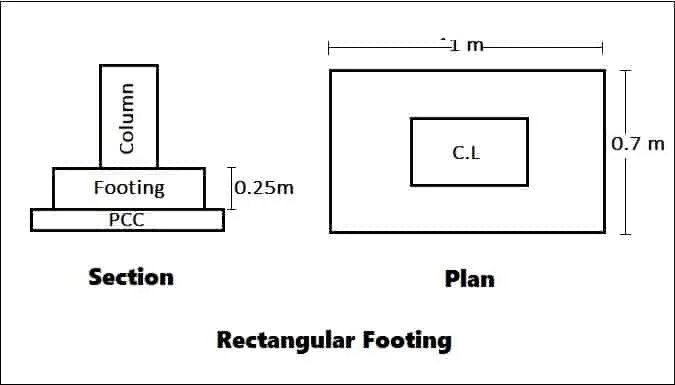## Register Now

Lorem ipsum dolor sit amet, consectetur adipiscing elit.Morbi adipiscing gravdio, sit amet suscipit risus ultrices eu.Fusce viverra neque at purus laoreet consequa.Vivamus vulputate posuere nisl quis consequat.

## How To Calculate Volume Of Concrete, Shuttering Area & Bitumen Paint Area For Plain Footing

In this article I will discuss how to calculate the volume of concrete, shuttering area and bitumen paint area for plain footing (Square footing & Rectangular footing).

## A. Square Footing:Given,

Length of footing = 0.5 m

Breadth of footing = 0.5 m

Depth of footing = 0.25 m

Size of column = 0.2 x 0.1

1. Volume of concrete = Area of sq. footing x Depth of sq footing

Area of sq. footing = L x B = 0.5 x 0.5 = 0.25 m²

Volume of concrete = 0.25 x 0.25 = 0.0625 m³.

2. Shuttering area = 2 (L + B) x D

= 2 (0.5+0.5) x 0.25

= 0.5m²

3. Bitumen paint area = Shuttering area + (Top area of footing – column area)

= 0.5 + [(0.5 x0.5) -(0.2 x 0.1)]

=0.5 + [0.25 – 0.2)]

= 0.73 m².

## 2. Rectangular Footing:Given,

Length of footing = 1 m

Breadth of footing = 0.7 m

Depth of footing = 0.25 m

Size of column = 0.4 x 0.3

1. Volume of concrete = Area of sq. footing x Depth of sq footing

Area of sq. footing = L x B = 1 x 0.7 = 0.7 m²

Volume of concrete = 0.7 x 0.25 = 0.175 m³.

2. Shuttering area = 2 (L + B) x D

= 2 (1+0.7) x 0.25

= 0.85m²

3. Bitumen paint area = Shuttering area + (Top area of footing – column area)

= 0.85 + [(1 x0.7) -(0.4 x 0.3)]

=0.85 + [0.7 – 0.12)]

= 1.43 m².

##### Also Read – HOW TO CALCULATE QUANTITY OF BINDING WIRES FOR STEEL BARS.

error: Content is protected !!